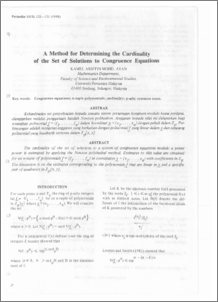# A Method for Determining the Cardinality of the Set of Solutions to Congruence Equations

## Citation

Mohd Atan, Kamel Ariffin (1988) A Method for Determining the Cardinality of the Set of Solutions to Congruence Equations. Pertanika, 11 (1). pp. 125-131.

## Abstract

The cardinality of the set of solutions to a system of congruence equations module a prime power is estimated by applying the Newton polyhedral method. Estimates to this value are obtained for an n-tuple of polynomials f = _ (f1, ... ,fn) in coordinates -f = (xl' ... ,xn) with coefficients in Zp. The discussion is 011 the estimates corresponding to the polynomials f that are linear in x and a specific pair of quadratics in Zp(x,y)Preview
PDF
A_Method_for_Determining_the_Cardinality.pdfView Item